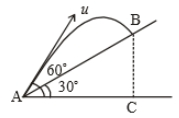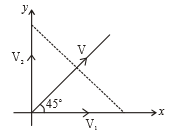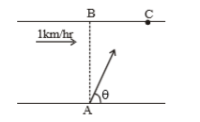Time taken by the projectile to reach from A to B is t. Then the distance AB is equal to :1. $\frac{ut}{\sqrt{3}}$

2. $\frac{\sqrt{3}ut}{2}$

3. $\sqrt{3}ut$

4. 2ut

Subtopic:  Projectile Motion |
To view explanation, please enrol in the course below.
NEET 2021 - Achiever Batch - Aryan Raj Singh

Difficulty Level:

A particle projected with kinetic energy ${\mathrm{k}}_{0}$ with an angle of projection $\mathrm{\theta }$. Then the variation of kinetic K with vertical displacement y is

1.  linear

2.  parabolic

3.  hyperbolic

4.  periodic

Subtopic:  Projectile Motion |
To view explanation, please enrol in the course below.
NEET 2021 - Achiever Batch - Aryan Raj Singh

Difficulty Level:

Three particles moving with constant velocities  and V respectively as given in the figure. After some time all three particles are in the same line, then relation among  and V is1.

2.

3.

4.

Subtopic:  Speed & Velocity |
To view explanation, please enrol in the course below.
NEET 2021 - Achiever Batch - Aryan Raj Singh

Difficulty Level:

A river is flowing with a speed of 1 km/hr. A swimmer wants to go to point 'C' starting from 'A'. He swims with a speed of 5 km/hr, at an angle $\theta$ w.r.t. the river. If AB=BC=400m. Then-(1) time taken by the man is 12 min

(2) time taken by the man is 8 min

(3) the value of $\theta$ is 45$°$

(4) the value of $\theta$ is 53$°$

Subtopic:  Relative Motion |
To view explanation, please enrol in the course below.
NEET 2021 - Achiever Batch - Aryan Raj Singh

Difficulty Level:

A body is thrown horizontally with a velocity $\sqrt{2gh}$ from the top of a tower of height h. It strikes the level ground through the foot of the tower at a distance x from the tower. The value of x is:

(1) h

(2) $\frac{h}{2}$

(3) 2h

(4) $\frac{2h}{3}$

Subtopic:  Projectile Motion |
To view explanation, please enrol in the course below.
NEET 2021 - Achiever Batch - Aryan Raj Singh

Difficulty Level:

A particle starts from the origin at t=0 and moves in the x-y plane with constant acceleration 'a' in the y direction. Its equation of motion is $y=b{x}^{2}$. The x component of its velocity (at t=0) is:

(1) variable

(2) $\sqrt{\frac{2a}{b}}$

(3) $\frac{a}{2b}$

(4) $\sqrt{\frac{a}{2b}}$

Subtopic:  Acceleration |
To view explanation, please enrol in the course below.
NEET 2021 - Achiever Batch - Aryan Raj Singh

Difficulty Level:

A body projected with velocity u with an angle of projection $\theta$. Change in velocity after the time (t) from the projection is:

(1) gt

(2) $\frac{1}{2}g{t}^{2}$

(3) u sin$\theta$

(4) u cos$\theta$

Subtopic:  Projectile Motion |
To view explanation, please enrol in the course below.
NEET 2021 - Achiever Batch - Aryan Raj Singh

Difficulty Level:

A boat is sent across a river in perpendicular direction with a velocity of 8 km/hr. If the resultant velocity of boat is 10 km/hr, then velocity of the river is :

(1) 10 km/hr

(2) 8 km/hr

(3) 6 km/hr

(4) 4 km/hr

Subtopic:  Relative Motion |
To view explanation, please enrol in the course below.
NEET 2021 - Achiever Batch - Aryan Raj Singh

Difficulty Level:

A boat is moving with velocity of $3\stackrel{^}{i}+4\stackrel{^}{j}$ in river and water is moving with a velocity of $-3\stackrel{^}{i}-4\stackrel{^}{j}$ with respect to ground. Relative velocity of boat with respect to water is :

(1) $-6\stackrel{^}{i}-8\stackrel{^}{j}$

(2) $6\stackrel{^}{i}+8\stackrel{^}{j}$

(3) $8\stackrel{^}{j}$

(4) $6\stackrel{^}{i}$

Subtopic:  Relative Motion |
To view explanation, please enrol in the course below.
NEET 2021 - Achiever Batch - Aryan Raj Singh

Difficulty Level:

A boat moves with a speed of 5 km/h relative to water in a river flowing with a speed of 3 km/h and having a width of 1 km. The minimum time taken around a round trip is

(1) 5 min

(2) 60 min

(3) 20 min

(4) 30 min

Subtopic:  Relative Motion |
To view explanation, please enrol in the course below.
NEET 2021 - Achiever Batch - Aryan Raj Singh

Difficulty Level: